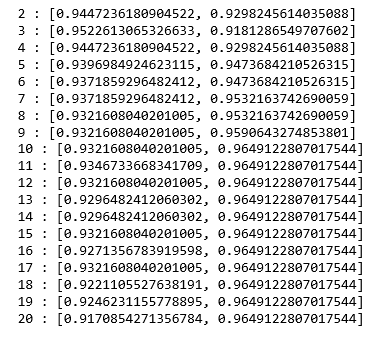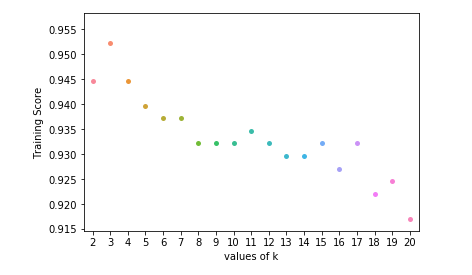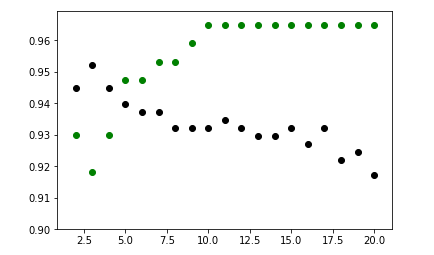# ML | Implementation of KNN classifier using Sklearn

Prerequisite: K-Nearest Neighbours Algorithm

K-Nearest Neighbors is one of the most basic yet essential classification algorithms in Machine Learning. It belongs to the supervised learning domain and finds intense application in pattern recognition, data mining and intrusion detection. It is widely disposable in real-life scenarios since it is non-parametric, meaning, it does not make any underlying assumptions about the distribution of data (as opposed to other algorithms such as GMM, which assume a Gaussian distribution of the given data).

This article will demonstrate how to implement the K-Nearest neighbors classifier algorithm using Sklearn library of Python.

Step 1: Importing the required Libraries

 `import` `numpy as np ` `import` `pandas as pd ` `from` `sklearn.model_selection ``import` `train_test_split ` `from` `sklearn.neighbors ``import` `KNeighborsClassifier ` `import` `matplotlib.pyplot as plt  ` `import` `seaborn as sns `

 `cd C:\Users\Dev\Desktop\Kaggle\Breast_Cancer ` `# Changing the read file location to the location of the file ` ` `  `df ``=` `pd.read_csv(``'data.csv'``) ` ` `  `y ``=` `df[``'diagnosis'``] ` `X ``=` `df.drop(``'diagnosis'``, axis ``=` `1``) ` `X ``=` `X.drop(``'Unnamed: 32'``, axis ``=` `1``) ` `X ``=` `X.drop(``'id'``, axis ``=` `1``) ` `# Separating the dependent and independent variable ` ` `  `X_train, X_test, y_train, y_test ``=` `train_test_split( ` `             ``X, y, test_size ``=` `0.3``, random_state ``=` `0``) ` `# Splitting the data into training and testing data `

Step 3: Training the model

 `K ``=` `[] ` `training ``=` `[] ` `test ``=` `[] ` `scores ``=` `{} ` ` `  `for` `k ``in` `range``(``2``, ``21``): ` `    ``clf ``=` `KNeighborsClassifier(n_neighbors ``=` `k) ` `    ``clf.fit(X_train, y_train) ` ` `  `    ``training_score ``=` `clf.score(X_train, y_train) ` `    ``test_score ``=` `clf.score(X_test, y_test) ` `    ``K.append(k) ` ` `  `    ``training.append(training_score) ` `    ``test.append(test_score) ` `    ``scores[k] ``=` `[training_score, test_score] `

Step 4: Evaluating the model

 `for` `keys, values ``in` `scores.items(): ` `    ``print``(keys, ``':'``, values) `We now try to find the optimum value for ‘k’ ie the number of nearest neighbors.

Step 5: Plotting the training and test scores graph

 `ax ``=` `sns.stripplot(K, training); ` `ax.``set``(xlabel ``=``'values of k'``, ylabel ``=``'Training Score'``)   ` ` `  `plt.show() ` `# function to show plot ``ax ``=` `sns.stripplot(K, test); ` `ax.``set``(xlabel ``=``'values of k'``, ylabel ``=``'Test Score'``) ` `plt.show() ``plt.scatter(K, training, color ``=``'k'``) ` `plt.scatter(K, test, color ``=``'g'``) ` `plt.show() ` `# For overlapping scatter plots `From the above scatter plot, we can come to the conclusion that the optimum value of k will be around 5.

My Personal Notes arrow_drop_upCheck out this Author's contributed articles.

If you like GeeksforGeeks and would like to contribute, you can also write an article using contribute.geeksforgeeks.org or mail your article to contribute@geeksforgeeks.org. See your article appearing on the GeeksforGeeks main page and help other Geeks.

Please Improve this article if you find anything incorrect by clicking on the "Improve Article" button below.

Improved By : shubham_singh

Article Tags :
Practice Tags :

Be the First to upvote.

Please write to us at contribute@geeksforgeeks.org to report any issue with the above content.